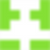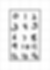# Identicons

We have seen them everywhere. From GitHub to Stack Overflow, identicons have been the face of users who do not have an associated Gravatar or a profile photo.I wanted to figure out how these were generated and came across this blog post by GitHub where they announced the introduction of identicons on their site.

Our Identicons are simple 5×5 “pixel” sprites that are generated using a hash of the user’s ID. The algorithm walks through the hash and turns pixels on or off depending on even or odd values. These generated patterns, combined with hash-determined color values, ensures a huge number of unique Identicons.

While the identicon itself is a 5x5 image, one can notice that it is symmetrical. The fourth and fifth columns mirror the second and first columns respectively. This implies that these identicons can be expressed as a 3x5 matrix. So, a 15 digit sequence is sufficient to represent an identicon. As for the color, we can use a 6 bit sequence from this 15 bit number itself and generate a color code in the hex format.

Let's try and build this now.

The 15 digit sequence has to be based on the given string and should be reproducible. Let's use hashing to solve this. We can use this example from MDN to generate the hash. The SHA-1 algorithm shouldn't be used for anything serious but since this is a fun little project, let's go with it.

We can use this example from MDN to generate the hash. The SHA-1 algorithm produces 40 character long hashes and for our simple fun reproduction, this should be sufficient. Being a non-cryptographic use case, we have the luxury of not worrying about collisions.

``````function hexString(buffer) {
let byteArray = new Uint8Array(buffer);

let hexCodes = [...byteArray].map(value => {
let hexCode = value.toString(16);
});

return hexCodes.join('');
}

function getHash(message) {
let encoder = new TextEncoder();
let data = encoder.encode(message);

return window.crypto.subtle.digest('SHA-1', data);
}

async function handleInput() {
let userInput = document.getElementById('message').value;
let hash = hexString(await getHash(userInput));

console.log(hash);  // logs f10e2821bbbea527ea02200352313bc059445190
}
``````

Now, we need to convert this 40 character long hash into a 15 bit number.In the above matrix, the value of `n` at position `(i, j)` is given by `i*3 + j`. We can then simply look at the `n`th position of the hash for `0 <= n <= 14`.

This can be represented in code as:

``````for (let i = 0; i < 3; i++) {
for (let j = 0; j < 3; j++) {
let n = hash.charAt(i*3 + j);
}
}
``````

To determine the mirrored values to fill the fourth and fifth columns, we can modify the above code to:

``````for (let i = 0; i < 5; i++) {
for (let j = 0; j < 5; j++) {
let J = j > 2 ? (4 - j) : j;
let n = hash.charAt(i*3 + J);
}
}
``````

Using the above code, we have arrived at the hash `f10e2821bbbea52`. We now need to find a way to use this hash to determine which of the boxes in the grid have to be filled. One trivial solution to this is to take each of these characters and check if it is divisible by 2 in the decimal form.

``````for (let i = 0; i < 5; i++) {
for (let j = 0; j < 5; j++) {
let J = j > 2 ? (4 - j) : j;
let n = hash.charAt(i*3 + J);
let shouldFill = parseInt(n, 16) % 2;
}
}
``````

All that's left is to determine the color of the fill. While we can just use a sequence of 6 digits from the hash `f10e2821bbbea52` that we generated above, let's use some of the extra characters from the 40 character long hash that we generated previously.

``````function getColor(hash) {
return `#\${hash.slice(-6)}`;
}

let color = getColor('f10e2821bbbea527ea02200352313bc059445190');
console.log(color);  // logs #445190
``````

Now putting this all together using some JavaScript and HTML Canvas, we get a working generator that produces identicons similar to the ones generated by GitHub.